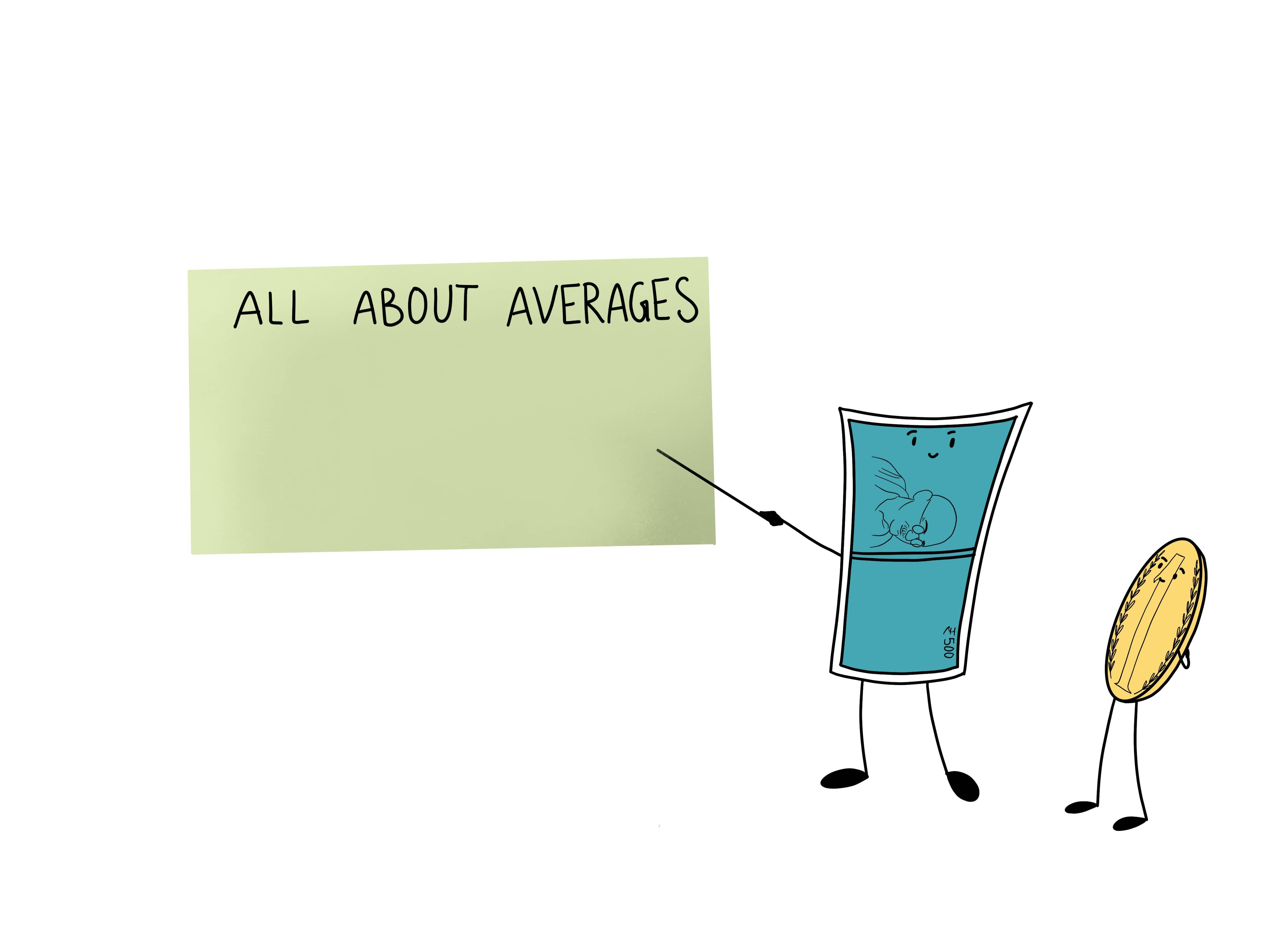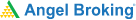# What is a Moving Average ? Explained in DetailWe have all learnt about averages in school, moving average is just an extension of that. Moving averages are trend indicators and are frequently used due to their simplicity and effectiveness.

Every keen-eyed investor and trader in a financial market makes his or her earnings by conducting intensive research and utilising crucial markers before every transaction. They are benefitted by processes such as technical analysis and the variety of indicators that help gauge the trends and movements within the market.

One such significant indicator, used by a variety of market participants, is the Moving Average. But what is Moving Average exactly? And how can you read and interpret it to make informed trading decisions? Let us explore the concept in detail.

## What is Moving Average?

Moving Average is essentially a technical indicator that is calculated as the average of a given set of data. In terms of technical analysis, this data is typically the various price points of a security, such as stocks or commodities. The Moving Average is thus calculated by adding up all the values of the data points of the security and dividing it with the total number of data points.

The indicator is known as a ‘moving’ average since its value keeps changing as the data, that is the underlying price values of the stock, keeps changing over time. When taken over a given period of time, such as 10 minutes or a week, the Moving Average represents the average value of the price action that the security underwent within that duration.

### Understanding Moving Averages

As an indicator, the Moving Average meaning is determined by the past price values of a security. It presents an average price of the asset over a period of time. Therefore, it can act as a useful indicator of where its price direction is headed for the future. It smoothes the price action and gives traders a clear idea of the overall price trend of the security.

If the Moving Average for a security is angled upwards, its price values are or have recently been rising. This denotes an upward trend. On the other hand, a downward angled Moving Average denotes a fall in price or a downtrend.

Moreover, since Moving Averages closely follow historical price movements, they can also help in establishing support and resistance levels. Most traders will follow the Moving Average to see if the price is going towards it, bouncing back or breaking the established resistance/support level. They also help traders in identifying potential entry and exit points into certain securities.

The length of Moving Averages can be changed based on the individual objectives of the trader. Therefore, short-term trading can make use of short Moving Averages, such as those extended across 30 days. On the other long-term investments can make use of long Moving Averages such as those extended over 200 days.

Learning & Earning is now super simple₹ 0 Equity Delivery

No Hidden Charges₹ 20 Per Order For Intraday

FAQ,Currencies & CommoditiesZERO Brokerage*

on ALL SegmentsFREE Margin

Trade Funding

### Types of Moving Averages

While Moving Averages serve as useful indicators for almost all market participants, not all of them use the same form of Moving Average. Overall, Moving Averages can be classified into three major types:

• Simple Moving Average: The most commonly used form of Moving Average in technical analysis is the Simple Moving Average. It is calculated by taking the mean of a set of values (mostly, prices of a security) and dividing it by the number of values. It can be calculated as follows:

(A1 + A2 + A3 + A4…An) / n = SMA

Where n is the number of time periods and A is the average within a given time period.

The most common periods for Simple Moving Average tracking are 8, 20, 5o, 100 and 200 days or periods.

• Exponential Moving Average: The other type of Moving Average is known as the Exponential Moving Average. This is a weighted form of calculating Moving Averages, whereby recent price values are given more weight than past price values. To achieve the accurate Exponential Moving Average Value, the trader must establish the Simple Moving Average Of the security prices first. This value then undergoes a formula that gives decreasing weightage to the average of each passing period.

Exponential Moving Averages adapt better and faster to changing price movements than Simple Moving Average.

#### Wrapping up

The Moving Average is a quick, straightforward and effective means for traders to develop an understanding of the current market trends. Since they are calculated by accounting for past price movements, Moving Averages are a popular tool for traders to predict future price directions before making a transaction. However, like all technical indicators, it is recommended that Moving Averages should be used in conjunction with other factors to form an accurate, overall analysis of the market opportunities.

#### A quick recap

• A moving average (MA) is a widely used technical indicator that smooths out price trends by filtering out the “noise” from random short-term price fluctuations.
• A standard average calculation is a quick approximation of a series of numbers
• In an average calculation where the latest data is included, and the oldest is excluded is called a Moving Average
• The simple moving average (SMA) gives equal weightage to all data points in the series
• An exponential moving average (EMA) scales the data according to its newness. Recent data gets the maximum weightage and the oldest gets the least weightageTest Your Knowledge

Take the quiz for this chapter & mark it complete.

Comments (0)

Add Comment

Get Information Mindfulness!

Catch-up With Market

News in 60 Seconds.The perfect starter to begin and stay tuned with your learning journey anytime and anywhere.Ready To Trade? Start withOpen an account